# Advanced Tutorial Lesson 10: Designing a Digital Ramp Generator

 Tutorial Project: Designing a Digital Ramp Generator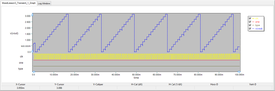Objective: In this project, the basic concepts of RF.Spice A/D are demonstrated, and a simple voltage divider is modeled and examined. Concepts/Features: Ripple Counter Toggle Flip-Flop Analog Switch Ladder D/a Converter Ramp Generator Minimum Version Required: All versions

## What You Will Learn

In this tutorial you will combine a ripple counter with a ladder D/A converter to build a ramp generator. You will also learn about voltage-controlled analog switches and will use them to select between up-ramp and down-ramp options.

## Building the Ripple Counter Using Toggle Flip-Flops

The following is a list of parts needed for this part of the tutorial lesson:

Part Name Part Type Part Value
HIGH Toggle Switch N/A
TYPE Toggle Switch N/A
A1 - A4 T-Type Flip-Flop Defaults
CLK Digital Clock Period = 1m, Pulse Width = 500u
R1 - R4 Resistor 2k
R5 - R7 Resistor 1k
R8 - R9 Resistor 2k
R10 Resistor 1k
Vcc DC Bias Voltage Source 15V
Vee DC Bias Voltage Source -15V
X1 LM741 Op-Amp Defaults
X2 - X5 Single-Pole Double-Throw (SPDT) Switch Defaults
VOUT Voltage Probe Marker N/A

In Digital Tutorial Lesson 4, you used JK flip-flops to build a 4-bit binary ripple counter. In this lesson, however, you will use four Toggle flip-flops for the same purpose. All the T pins of the four flip-flops are set to "high" using a toggle switch. The digital clock signal is input to the first (LSB) flip-flop, while the clock of all the other flip-flops is fed by the Q-output of the previous stage. The four outputs of the binary counter generate all the sixteen 4-bit binary numbers 0000, 0001, 0010, ..., 1110, 1111, in a cyclical manner. Connect the relevant parts as shown in the figure below: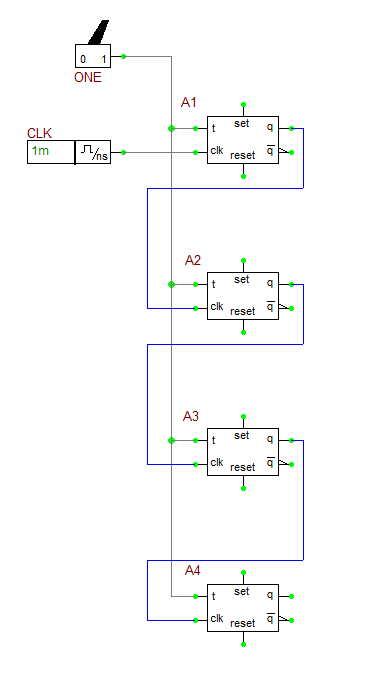The 4-bit ripple counter made of four Toggle flip-flops.

## Building the Ladder D/A Converter

The 4-bit binary words generated by the four digital inputs at each clock cycle correspond to the analog voltage levels 0V, 210mV, 420mV, ..., 2.94V, 3.15V, respectively, or 0.21k V in general, where k = 0, 1, 2, ..., 15. Build the four-input ladder D/A converter circuit shown in the figure below using the Op-Amp. This is identical to the R-2R ladder circuit of the last tutorial lesson except for the missing digital inputs, clock and digital control gates. Note that four outputs of the ripple counter are already clocked. Of you take the Q_bar outputs of the four T flip-flops and feed them directly to the ladder D/A converter, the resulting analog output will successively increase from 0 to 3.15V with increments of 210mV at each clock cycle. Then, it drops down to zero and the discrete ramp starts all over again. In other words, you will have an Up-Ramp. On the other hand, if you take the Q outputs of the four T flip-flops, you will get a Down-Ramp.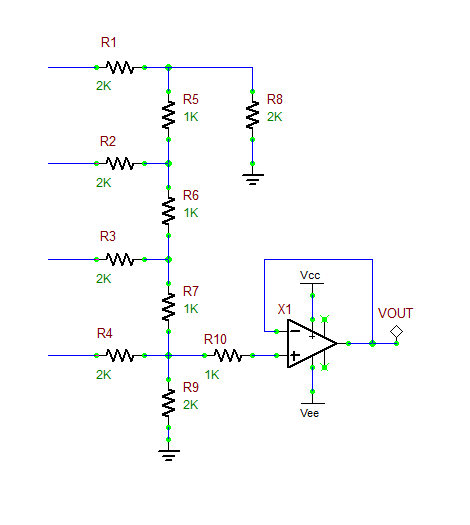The R-2R Ladder D/A converter circuit without the digital control.

## Building the Switching Network

To have both options of Up-Ramp and Down-Ramp, you need to build a switching network than can select between the Q and Q_bar output of each T flip-flop corresponding to each binary bit. In this project, you will use a single-pole double-throw (SPDT) analog switch, which you can access from Menu > Parts > Analog Switches. This switch is a 5-pin device and selects between two outputs depending on its control voltage. When the control voltage is equal to or less than the turn-off voltage "voff" or at "0/low" state, the output is thrown at the "0" pin. When the control voltage is equal to or greater than the turn-on voltage "von" or at "1/high" state, the output is thrown at the "1" pin. The default values of vin and voff are 3.3V and 0V, respectively. Since in this project, you are using mixed-mode circuits with RF.Spice's default 5V high state voltage, you can keep the default values of the SPDT switch as they are adequate for our purpose.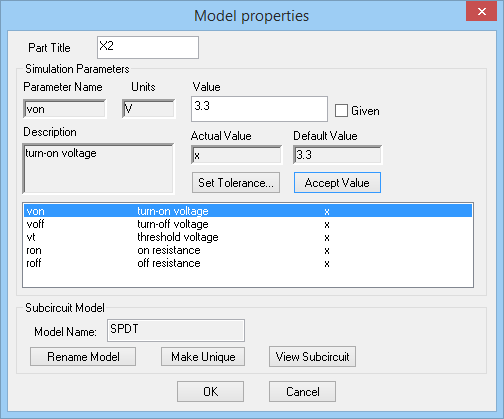The property dialog of the analog SPDT switch.

Since each SPDT switch has to select between Q and Q-bar outputs of a T flip-flop on the left, you need to flip the four SPDT switch horizontally using the keyboard shortcut Ctrl+F. You can also rotate them twice clockwise right after placing each part, while they are still in place/edit mode. The figure below shows how you should place and connect the four SPDT switches. The control voltage pin of all four switches are connected directly to the digital toggle switch called "TYPE".The switching network made up of four analog SPDT switches.

## Putting It All Together and Testing the Ramp Generator

Connect the three subcircuits together as shown in the figure below:

Before running a simulation, you need to change some SPICE simulation options related to the mixed-mode circuits. Since you have used the default settings of the toggle flip-flops, their default rise and fall delay times (tPLH and tPHL) are 1ns. Open the SPICE Simulation Options Dialog and change the default values of two parameters: Low-to-High delay for Implicit D-to-A Converters (XSPICE) and High-to-Low delay for Implicit D-to-A Converters (XSPICE) to 5ns. This will allow RF.Spice's implicit D/A converters to be able to handle the digital output signals from the flip-flops.Changing mixed-mode parameters in the SPICE Simulation Options dialog.

Set the period and pulse width of the digital clock CLK set to 1ms and 500μs, respectively. Set the "TYPE" switch initially to the "0" position. Run a Transient Test of the ramp generator with the following parameters:

 Start Time 0 100m 1u 1u Defaults

You need 16 clock cycles to cover all the counter states. Therefore, the period of the ramp generator is T = 16ΔT = 16ms, and its frequency is f0 = 1/(16ΔT) = 1/(16ms) = 62.5Hz, where ΔT is the clock period. The output voltage graph is shown in the figure below.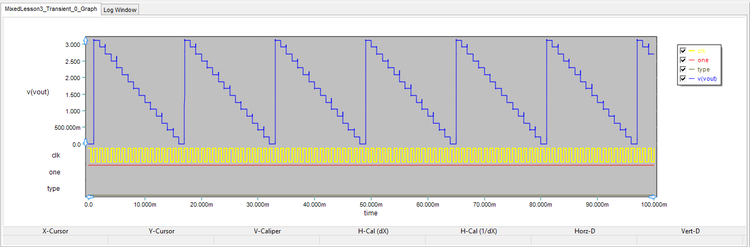The graph of output voltage of the ramp generator when TYPE = 0 (Down-Ramp).

Next, toggle the TYPE switch to the "1" position and repeat the transient test and observe the output voltage of your ramp generator as shown below: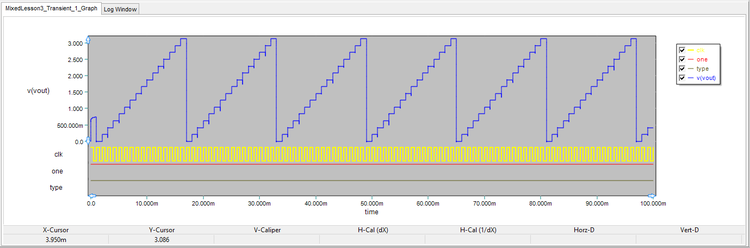The graph of output voltage of the ramp generator when TYPE = 1 (Up-Ramp).